# 1.02.Mathematical_background.pptx

29. May 2023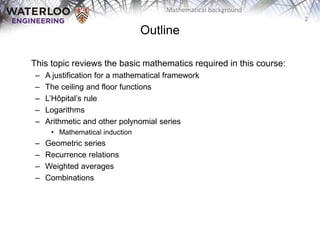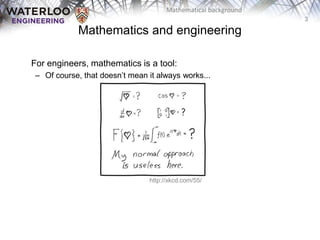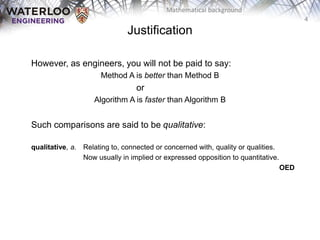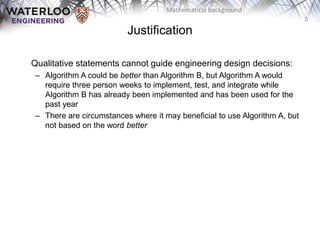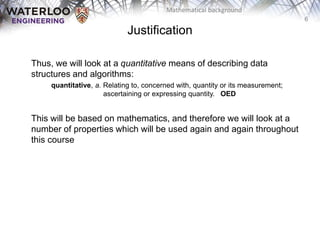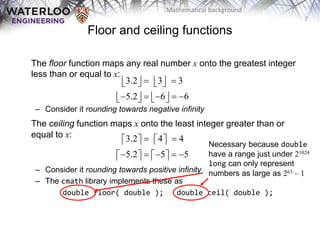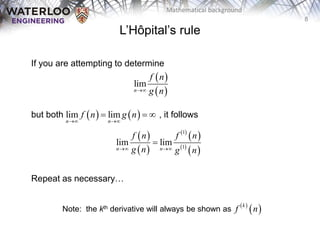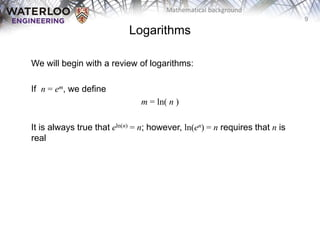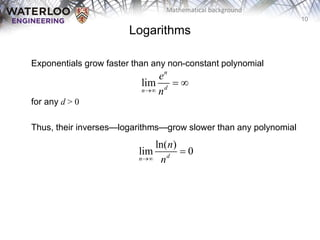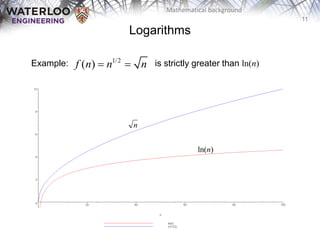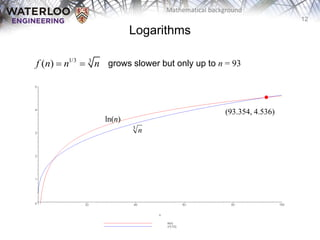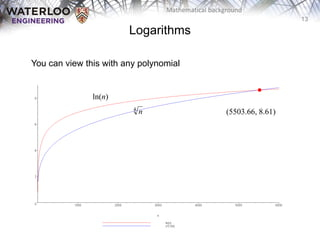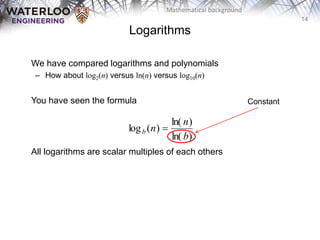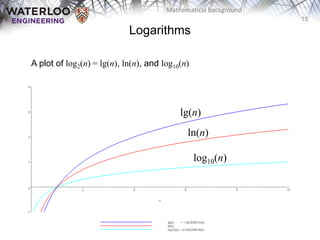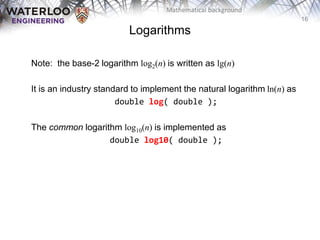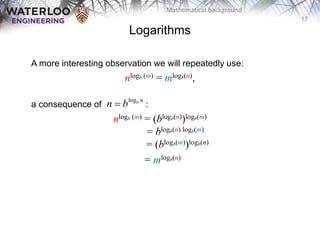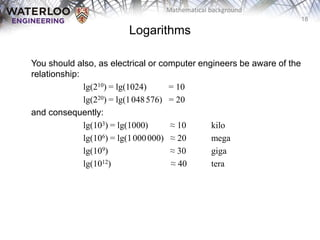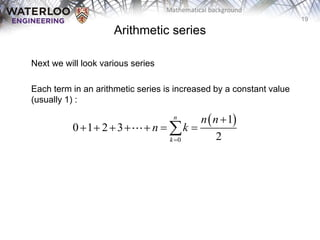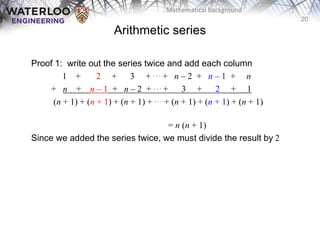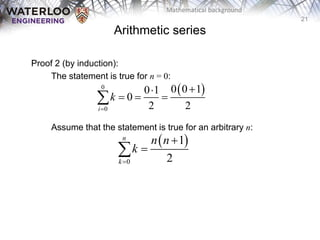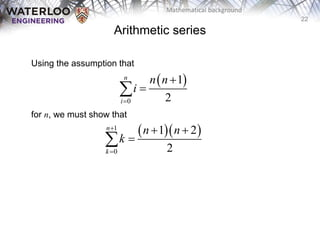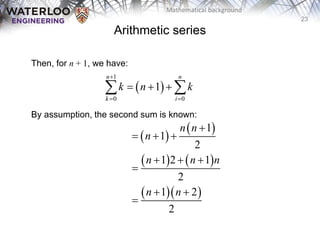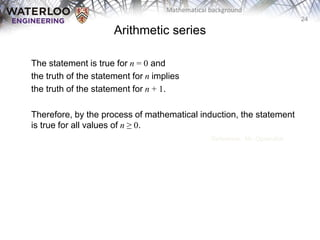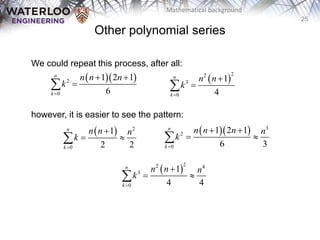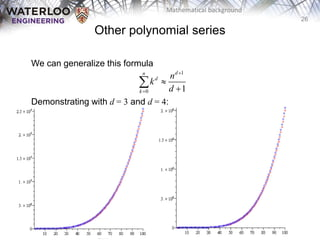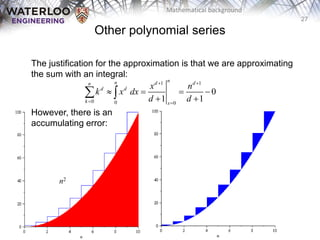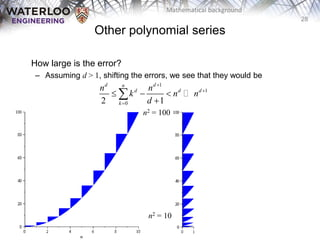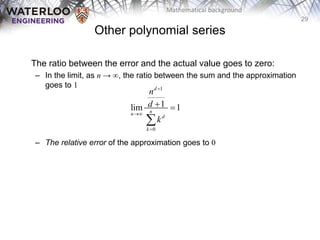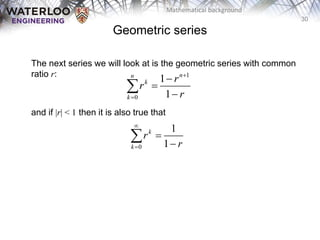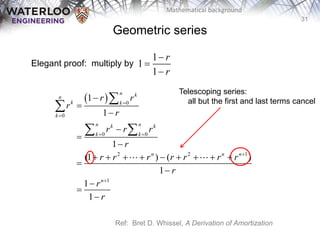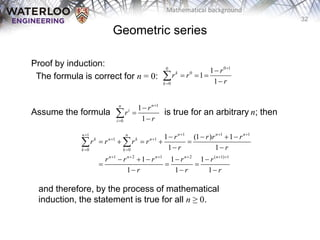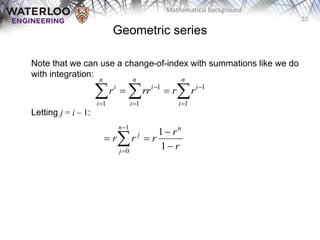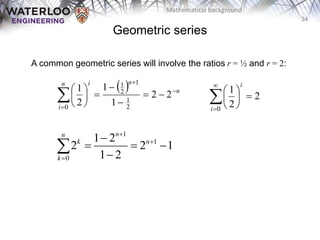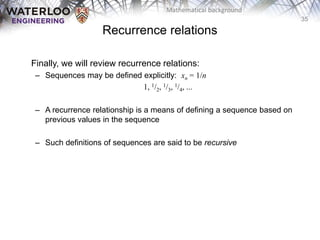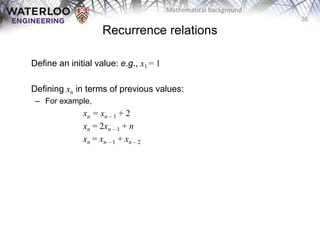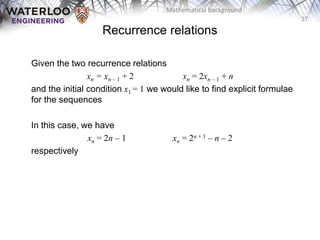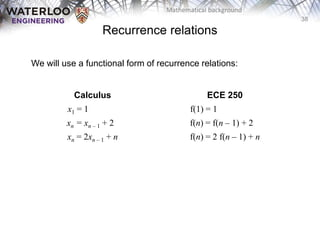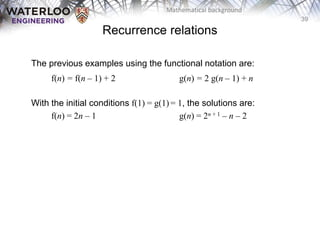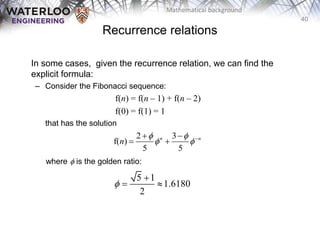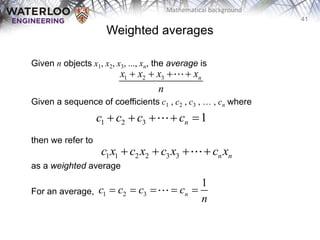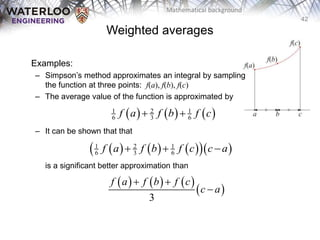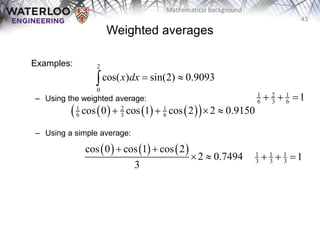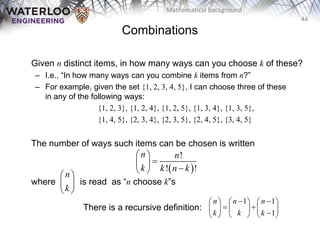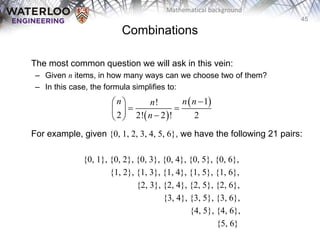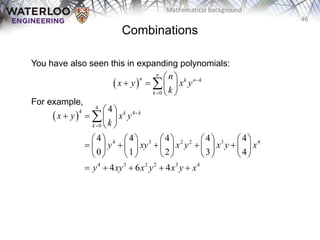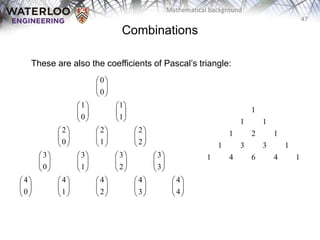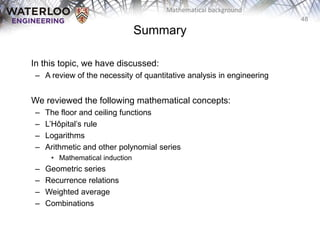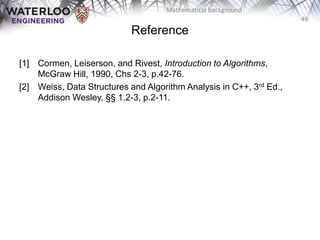1 von 50

### 1.02.Mathematical_background.pptx

• 1. ECE 250 Algorithms and Data Structures Douglas Wilhelm Harder, M.Math. LEL Department of Electrical and Computer Engineering University of Waterloo Waterloo, Ontario, Canada ece.uwaterloo.ca dwharder@gmail.com © 2006-2018 by Douglas Wilhelm Harder. Some rights reserved. Mathematical background
• 2. 2 Mathematical background This topic reviews the basic mathematics required in this course: – A justification for a mathematical framework – The ceiling and floor functions – L’Hôpital’s rule – Logarithms – Arithmetic and other polynomial series • Mathematical induction – Geometric series – Recurrence relations – Weighted averages – Combinations Outline
• 3. 3 Mathematical background Mathematics and engineering For engineers, mathematics is a tool: – Of course, that doesn’t mean it always works... http://xkcd.com/55/
• 4. 4 Mathematical background Justification However, as engineers, you will not be paid to say: Method A is better than Method B or Algorithm A is faster than Algorithm B Such comparisons are said to be qualitative: qualitative, a. Relating to, connected or concerned with, quality or qualities. Now usually in implied or expressed opposition to quantitative. OED
• 5. 5 Mathematical background Qualitative statements cannot guide engineering design decisions: – Algorithm A could be better than Algorithm B, but Algorithm A would require three person weeks to implement, test, and integrate while Algorithm B has already been implemented and has been used for the past year – There are circumstances where it may beneficial to use Algorithm A, but not based on the word better Justification
• 6. 6 Mathematical background Thus, we will look at a quantitative means of describing data structures and algorithms: quantitative, a. Relating to, concerned with, quantity or its measurement; ascertaining or expressing quantity. OED This will be based on mathematics, and therefore we will look at a number of properties which will be used again and again throughout this course Justification
• 7. 7 Mathematical background Floor and ceiling functions The floor function maps any real number x onto the greatest integer less than or equal to x: – Consider it rounding towards negative infinity The ceiling function maps x onto the least integer greater than or equal to x: – Consider it rounding towards positive infinity – The cmath library implements these as double floor( double ); double ceil( double ); 3.2 3 3 5.2 6 6                        3.2 4 4 5.2 5 5                        Necessary because double have a range just under 21024 long can only represent numbers as large as 263 – 1
• 8. 8 Mathematical background L’Hôpital’s rule If you are attempting to determine but both , it follows Repeat as necessary…     lim n f n g n      lim lim n n f n g n                  1 1 lim lim n n f n f n g n g n        k f n Note: the kth derivative will always be shown as
• 9. 9 Mathematical background We will begin with a review of logarithms: If n = em, we define m = ln( n ) It is always true that eln(n) = n; however, ln(en) = n requires that n is real Logarithms
• 10. 10 Mathematical background Exponentials grow faster than any non-constant polynomial for any d > 0 Thus, their inverses—logarithms—grow slower than any polynomial Logarithms lim n d n e n    ln( ) lim 0 d n n n  
• 11. 11 Mathematical background Example: is strictly greater than ln(n) 1/2 ( ) f n n n   ln(n) n Logarithms
• 12. 12 Mathematical background Logarithms grows slower but only up to n = 93 (93.354, 4.536) ln(n) 3 n 1/3 3 ( ) f n n n  
• 13. 13 Mathematical background You can view this with any polynomial ln(n) 4 n Logarithms (5503.66, 8.61)
• 14. 14 Mathematical background We have compared logarithms and polynomials – How about log2(n) versus ln(n) versus log10(n) You have seen the formula All logarithms are scalar multiples of each others ) ln( ) ln( ) ( log b n n b  Logarithms Constant
• 15. 15 Mathematical background A plot of log2(n) = lg(n), ln(n), and log10(n) lg(n) ln(n) log10(n) Logarithms
• 16. 16 Mathematical background Note: the base-2 logarithm log2(n) is written as lg(n) It is an industry standard to implement the natural logarithm ln(n) as double log( double ); The common logarithm log10(n) is implemented as double log10( double ); Logarithms
• 17. 17 Mathematical background A more interesting observation we will repeatedly use: nlogb (m) = mlogb(n), a consequence of : nlogb (m) = (blogb(n))logb(m) = blogb(n) logb(m) = (blogb(m))logb(n) = mlogb(n) Logarithms logb n n b 
• 18. 18 Mathematical background You should also, as electrical or computer engineers be aware of the relationship: lg(210) = lg(1024) = 10 lg(220) = lg(1048576) = 20 and consequently: lg(103) = lg(1000) ≈ 10 kilo lg(106) = lg(1000000) ≈ 20 mega lg(109) ≈ 30 giga lg(1012) ≈ 40 tera Logarithms
• 19. 19 Mathematical background Next we will look various series Each term in an arithmetic series is increased by a constant value (usually 1) :   0 1 0 1 2 3 2 n k n n n k           Arithmetic series
• 20. 20 Mathematical background Proof 1: write out the series twice and add each column 1 + 2 + 3 + . . . + n – 2 + n – 1 + n + n + n – 1 + n – 2 + . . . + 3 + 2 + 1 (n + 1) + (n + 1) + (n + 1) + . . . + (n + 1) + (n + 1) + (n + 1) = n (n + 1) Since we added the series twice, we must divide the result by 2 Arithmetic series
• 21. 21 Mathematical background Proof 2 (by induction): The statement is true for n = 0: Assume that the statement is true for an arbitrary n:   0 0 0 0 1 0 1 0 2 2 i k          0 1 2 n k n n k     Arithmetic series
• 22. 22 Mathematical background Using the assumption that for n, we must show that   0 1 2 n i n n i        1 0 1 2 2 n k n n k       Arithmetic series
• 23. 23 Mathematical background Then, for n + 1, we have: By assumption, the second sum is known:   1 0 0 1 n n k i k n k                    1 1 2 1 2 1 2 1 2 2 n n n n n n n n            Arithmetic series
• 24. 24 Mathematical background The statement is true for n = 0 and the truth of the statement for n implies the truth of the statement for n + 1. Therefore, by the process of mathematical induction, the statement is true for all values of n ≥ 0. Reference: Mr. Oprendick Arithmetic series
• 25. 25 Mathematical background We could repeat this process, after all: however, it is easier to see the pattern:   2 2 3 0 1 4 n k n n k        3 2 0 1 2 1 6 3 n k n n n n k          2 0 1 2 1 6 n k n n n k        2 2 4 3 0 1 4 4 n k n n n k      Other polynomial series   2 0 1 2 2 n k n n n k     
• 26. 26 Mathematical background We can generalize this formula Demonstrating with d = 3 and d = 4: 1 0 1 d n d k n k d      Other polynomial series
• 27. 27 Mathematical background The justification for the approximation is that we are approximating the sum with an integral: However, there is an accumulating error: 1 1 0 0 0 0 1 1 n n d d n d d k x x n k x dx d d             Other polynomial series n2
• 28. 28 Mathematical background How large is the error? – Assuming d > 1, shifting the errors, we see that they would be Other polynomial series 1 1 0 2 1 d d n d d d k n n k n n d         n2 = 100 n2 = 10
• 29. 29 Mathematical background The ratio between the error and the actual value goes to zero: – In the limit, as n → ∞, the ratio between the sum and the approximation goes to 1 – The relative error of the approximation goes to 0 Other polynomial series 1 0 1 lim 1 d n n d k n d k      
• 30. 30 Mathematical background The next series we will look at is the geometric series with common ratio r: and if |r| < 1 then it is also true that 1 0 1 1 n n k k r r r       0 1 1 k k r r      Geometric series
• 31. 31 Mathematical background Elegant proof: multiply by Ref: Bret D. Whissel, A Derivation of Amortization   0 0 0 0 2 2 1 1 1 1 1 (1 ) ( ) 1 1 1 n k n k k k n n k k k k n n n n r r r r r r r r r r r r r r r r r r                               Geometric series 1 1 1 r r    Telescoping series: all but the first and last terms cancel
• 32. 32 Mathematical background Proof by induction: The formula is correct for n = 0: Assume the formula is true for an arbitrary n; then and therefore, by the process of mathematical induction, the statement is true for all n ≥ 0. 0 1 0 0 0 1 1 1 k k r r r r         1 0 1 1 n n i i r r r       1 1 1 1 1 1 0 0 1 2 1 2 ( 1) 1 1 (1 ) 1 1 1 1 1 1 1 1 1 n n n n n k n k n k k n n n n n r r r r r r r r r r r r r r r r r r                                       Geometric series
• 33. 33 Mathematical background Note that we can use a change-of-index with summations like we do with integration: Letting j = i – 1:           n i i n i i n i i r r rr r 1 1 1 1 1 r r r r r n n j j        1 1 1 0 Geometric series
• 34. 34 Mathematical background A common geometric series will involve the ratios r = ½ and r = 2:   n n n i i                2 2 1 1 2 1 2 1 1 2 1 0 1 1 0 1 2 2 2 1 1 2 n n k n k          2 2 1 0           i i Geometric series
• 35. 35 Mathematical background Finally, we will review recurrence relations: – Sequences may be defined explicitly: xn = 1/n 1, 1/2, 1/3, 1/4, ... – A recurrence relationship is a means of defining a sequence based on previous values in the sequence – Such definitions of sequences are said to be recursive Recurrence relations
• 36. 36 Mathematical background Define an initial value: e.g., x1 = 1 Defining xn in terms of previous values: – For example, xn = xn – 1 + 2 xn = 2xn – 1 + n xn = xn – 1 + xn – 2 Recurrence relations
• 37. 37 Mathematical background Given the two recurrence relations xn = xn – 1 + 2 xn = 2xn – 1 + n and the initial condition x1 = 1 we would like to find explicit formulae for the sequences In this case, we have xn = 2n – 1 xn = 2n + 1 – n – 2 respectively Recurrence relations
• 38. 38 Mathematical background We will use a functional form of recurrence relations: Calculus ECE 250 x1 = 1............. f(1) = 1................... xn = xn – 1 + 2.. f(n) = f(n – 1) + 2... xn = 2xn – 1 + n f(n) = 2 f(n – 1) + n Recurrence relations
• 39. 39 Mathematical background The previous examples using the functional notation are: f(n) = f(n – 1) + 2 g(n) = 2 g(n – 1) + n With the initial conditions f(1) = g(1) = 1, the solutions are: f(n) = 2n – 1 g(n) = 2n + 1 – n – 2 Recurrence relations
• 40. 40 Mathematical background In some cases, given the recurrence relation, we can find the explicit formula: – Consider the Fibonacci sequence: f(n) = f(n – 1) + f(n – 2) f(0) = f(1) = 1 that has the solution where f is the golden ratio: 2 3 f( ) 5 5 n n n f f f f     5 1 1.6180 2 f    Recurrence relations
• 41. 41 Mathematical background Weighted averages Given n objects x1, x2, x3, ..., xn, the average is Given a sequence of coefficients c1 , c2 , c3 , … , cn where then we refer to as a weighted average For an average, 1 2 3 n x x x x n     1 2 3 1 n c c c c      1 1 2 2 3 3 n n c x c x c x c x     1 2 3 1 n c c c c n     
• 42. 42 Mathematical background Weighted averages Examples: – Simpson’s method approximates an integral by sampling the function at three points: f(a), f(b), f(c) – The average value of the function is approximated by – It can be shown that that is a significant better approximation than       1 2 1 6 3 6 f a f b f c            1 2 1 6 3 6 f a f b f c c a            3 f a f b f c c a   
• 43. 43 Mathematical background Weighted averages Examples: – Using the weighted average: – Using a simple average:         1 2 1 6 3 6 cos 0 cos 1 cos 2 2 0.9150     2 0 cos( ) sin(2) 0.9093 x dx          cos 0 cos 1 cos 2 2 0.7494 3     1 2 1 6 3 6 1    1 1 1 3 3 3 1   
• 44. 44 Mathematical background Combinations Given n distinct items, in how many ways can you choose k of these? – I.e., “In how many ways can you combine k items from n?” – For example, given the set {1, 2, 3, 4, 5}, I can choose three of these in any of the following ways: {1, 2, 3}, {1, 2, 4}, {1, 2, 5}, {1, 3, 4}, {1, 3, 5}, {1, 4, 5}, {2, 3, 4}, {2, 3, 5}, {2, 4, 5}, {3, 4, 5}, The number of ways such items can be chosen is written where is read as “n choose k”s There is a recursive definition:   ! ! ! n n k k n k         n k       1 1 1 n n n k k k                       
• 45. 45 Mathematical background Combinations The most common question we will ask in this vein: – Given n items, in how many ways can we choose two of them? – In this case, the formula simplifies to: For example, given {0, 1, 2, 3, 4, 5, 6}, we have the following 21 pairs: {0, 1}, {0, 2}, {0, 3}, {0, 4}, {0, 5}, {0, 6}, {1, 2}, {1, 3}, {1, 4}, {1, 5}, {1, 6}, {2, 3}, {2, 4}, {2, 5}, {2, 6}, {3, 4}, {3, 5}, {3, 6}, {4, 5}, {4, 6}, {5, 6}     1 ! 2 2! 2 ! 2 n n n n n          
• 46. 46 Mathematical background Combinations You have also seen this in expanding polynomials: For example,   0 n n k n k k n x y x y k              4 4 4 0 4 3 2 2 3 4 4 3 2 2 3 4 4 4 4 4 4 4 0 1 2 3 4 4 6 4 k k k x y x y k y xy x y x y x y xy x y x y x                                                   
• 47. 47 Mathematical background Combinations These are also the coefficients of Pascal’s triangle: 0 0 1 1 0 1 2 2 2 0 1 2 3 3 3 3 0 1 2 3 4 4 4 4 4 0 1 2 3 4                                                                                           1 1 1 1 2 1 1 3 3 1 1 4 6 4 1
• 48. 48 Mathematical background In this topic, we have discussed: – A review of the necessity of quantitative analysis in engineering We reviewed the following mathematical concepts: – The floor and ceiling functions – L’Hôpital’s rule – Logarithms – Arithmetic and other polynomial series • Mathematical induction – Geometric series – Recurrence relations – Weighted average – Combinations Summary
• 49. 49 Mathematical background  Cormen, Leiserson, and Rivest, Introduction to Algorithms, McGraw Hill, 1990, Chs 2-3, p.42-76.  Weiss, Data Structures and Algorithm Analysis in C++, 3rd Ed., Addison Wesley, §§ 1.2-3, p.2-11. Reference
• 50. 50 Mathematical background Usage Notes • These slides are made publicly available on the web for anyone to use • If you choose to use them, or a part thereof, for a course at another institution, I ask only three things: – that you inform me that you are using the slides, – that you acknowledge my work, and – that you alert me of any mistakes which I made or changes which you make, and allow me the option of incorporating such changes (with an acknowledgment) in my set of slides Sincerely, Douglas Wilhelm Harder, MMath dwharder@alumni.uwaterloo.ca